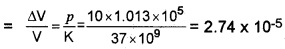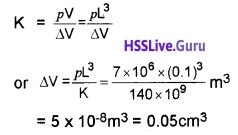# Plus One Physics Chapter Wise Questions and Answers Chapter 9 Mechanical Properties of Solids

Students can Download Chapter 9 Mechanical Properties of Solids Questions and Answers, Plus One Physics Chapter Wise Questions and Answers helps you to revise the complete Kerala State Syllabus and score more marks in your examinations.

## Kerala Plus One Physics Chapter Wise Questions and Answers Chapter 9 Mechanical Properties of Solids

### Plus One Physics Mechanical Properties of Solids One Mark Questions and Answers

Plus One Physics Mechanical Properties Of Solids Chapter 9 Question 1.
If longitudinal strain fora wire is 0.03 and its poisson’s ratio is 0.5, then its lateral strain is
(a) 0.003
(b) 0.0075
(c) 0.015
(d) 0.4
(c) 0.015
Poisson’s ratio σ = $$\frac { Lateral\quad strain }{ Longitudina\quad strain }$$
Lateral strain = Longitudinal strain × σ
= 0.03 × 0.5 = 0.015.

Mechanical Properties Of Solids Question Bank Chapter 9 Question 2.
Between steel and diamond, which is more elastic?
Steel.

Mechanical Properties Of Solids Important Questions Chapter 9 Question 3.
What is the value of Y for a perfectly elastic body?
Infinity.

Mechanical Properties Of Solids Neet Questions And Answers Pdf Chapter 9 Question 4.
Young’s modulus of the wire depends on
(a) length of the wire
(b) diameter of the wire
(c) material of the wire
(d) mass hanging from the wire
(c) material of the wire
Young’s modulus of wire depends only on the nature of the material of the wire.

Mechanical Properties Of Solids Class 11 Important Questions Pdf Question 5.
Two solid spheres of the same material have the same radius but one is hollow while the other is solid. Both spheres are heated to same temperature. Then
(a) the solid sphere expands more
(b) the hollow sphere expands more
(c) expansion is same for both
(d) nothing can be solid about their relative expansion if their masses are not given
(c) expansion is same for both.

Plus One Physics Chapter Wise Questions And Answers Chapter 9 Question 6.
For most materials the Young’s modulus is n times the rigidity modulus, where n is
(a) 2
(b) 3
(c) 4
(d) 5
(b) 3
For most materials, the modulus of rigidity, η is one third of the Young’s modulus, Y.
η = $$\frac{1}{3}$$Y or Y = 3η
∴ n = 3.

Hsslive Plus One Physics Chapter Wise Questions And Answers Chapter 9 Question 7.
The stress required to double the length of a wire of Young’s modulus Y is
(a) Y/2
(b) 2Y
(c) Y
(d) 4Y
(c) Let L be the length of a wire.
If the length of a wire is doubled, the longitudinal strain will be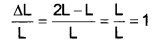Young’s modulus Y = $$\frac{\text { Stress }}{\text { Strain }}$$
∴ Y = Stress (∵ Strain = 1).

Plus One Physics Chapter Wise Questions And Answers Pdf Hsslive Chapter 9 Question 8.
What is the bulk modulus of a perfectly rigid body?
Infinity

Mechanical Properties Of Solids Neet Questions Chapter 9 Question 9.
What is shear modulus of liquid?
Zero

Mechanical Properties Of Solids Pdf Chapter 9 Question 10.
What is rigidity modulus of liquid?
Zero.

Mechanical Properties Of Solids Class 11 Questions And Answers Pdf Question 11.
Identify the type of modulus in twisting of cylinder.
Shear modulus

Plus One Physics Important Questions And Answers Pdf Chapter 9 Question 12.
Why work is required to be done to stretch a wire?
Work is required to be done against the inter molecular forces of attraction.

Mechanical Properties Of Solids Questions And Answers Chapter 9 Question 13.
Name the material which is famous for a large elastic after effect.
Glass

### Plus One Physics Mechanical Properties of Solids Two Mark Questions and Answers

Mechanical Properties Of Solids Neet Questions Pdf Chapter 9 Question 1.
Find stress required to double the length of a wire of Young’s modulus Y
Let L be the length of a wire.
If the length of a wire is doubled, the longitudinal strain will be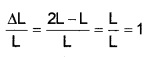Young’s modulus Y = $$\frac{\text { Stress }}{\text { Strain }}$$
∴ Y = Stress (∵ Strain = 1).

Hss Live Plus One Physics Chapter Wise Questions And Answers Chapter 9 Question 2.
When wire is bent back and forth, it becomes hot. Why?
When wire is bent back and forth, the deformations are beyond elastic limit. A part of work done is converted in to heat energy. Hence wire becomes hot.

### Plus One Physics Mechanical Properties of Solids Three Mark Questions and Answers

Class 11 Physics Chapter Mechanical Properties Of Solids Chapter 9 Question 1.
The stress strain graph of two bodies A and B are given in the figure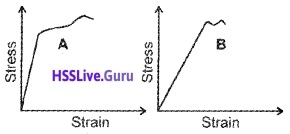1. Which of the material has greater youngs modulus?
2. Which of the two material is preferable to be used as a rope in a crane?
3. Redraw the graph and mark the regions in the graphs where the elastic force is strictly conservative

1. Youngs modulus, Y = $$\frac{\text { Stress }}{\text { Strain }}$$
The slope of above graph gives youngs modulus. The body A has larger slope. Hence youngs modulus of ‘A ‘is greater than ‘B’.

2. The fracture point is greater for A. Hence A is stronger. Due to high strength, the material A is used as rope in a crane.

3.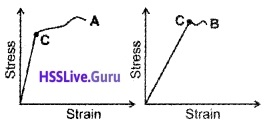Up to the point C, the elastic force is strictly conservative.

Mechanical Properties Of Solids Solutions Chapter 9 Question 2.
Hook’s law is said to be that fundamental law in elasticity.

1. State Hook’s law of Elasticity.
2. Name the different types of modulus of elasticity with their equations.

1. For small deformations, the stress and strain are proportional to each other, ie: stress ∝ strain stress = k × strain. Where k is the proportionality constant and is known as modulus of elasticity.

2.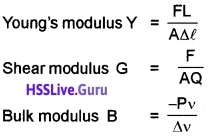### Plus One Physics Mechanical Properties of Solids Four Mark Questions and Answers

Mechanical Properties Of Solids Class 11 Solutions Chapter 9 Question 1.
When an external force deforms a solid, internal restoring forces are developed in the body giving rise to stress and strain.

1. Define stress and strain.
2. Draw the stress-strain diagram and mark the positions of elastic limit and the regions of elastic and plastic behaviors.

1. Stress: It is the restoring force developed per unit area of cross section.
Strain: The ratio of change in dimension to original dimension is called strain.

2.### Plus One Physics Mechanical Properties of Solids Five Mark Questions and Answers

Plus One Physics Chapter Wise Questions And Answers Pdf Chapter 9 Question 1.

1. Distinguish between perfectly plastic and perfectly elastic materials.
2. What is the quantity obtained from the slope of a stress-strain graph and what is the area under the curve?
3. Two wires have their lengths in the ratio 1:3 and radii in the ratio 2:1. What will be the ratio of elongations for the same linear stress?

1. When deforming forces are applied on a body its volume or shape will change. On removing the deforming forces it regains its original size and shape. It is called elastic body.

On removing the deforming forces. The body which cannot retain the original shape and size is called plastic body.

2. Modulus of elasticity. Area under stress strain graph gives workdone.

3. we know l1: l2, 1 : 3
r2 : r1, 2 : 1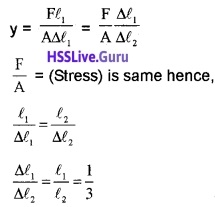Mechanical Properties Of Solids Questions Pdf Chapter 9 Question 2.
The modulus of elasticity of rubber is higher than that of steel.

1. Do you agree with this statement? Why?
3. Prove that the elastic potential energy density of a stretched wire is half the product of stress and strain.

1. No

2. Y = $$\frac{F l}{A \Delta l}$$
∆l is higher for rubber than steel. Hence youngs modulus is smaller for rubber than steel.

3. Workdone in stretching a wire.
= $$\frac{1}{2}$$ × stretching force × extension
= $$\frac{1}{2}$$ Fe
Let ‘a’ be the area of cross section and ‘L’ the length of the wire. The volume ‘aL’
Workdone for a volume ‘aL’ = $$\frac{1}{2}$$
Workdone per unit volume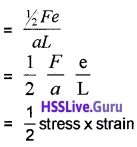Important Questions From Mechanical Properties Of Solids Class 11 Question 3.
We have common experience that a thin thread breakes if pulled at its ends. Also a thin metallic wire, when pulled at the ends elongates and then contracts, when released.

1. What do you mean by elasticity?
2. Draw a the stress-strain graph of a metal and mark elastic limit, plastic region, and fracture point.
3. A lift is tied with thick iron wires and its mass is 1000kg. What should be the minimum diameter of the wire if the maximum acceleration of the lift is 1.2 m/s2 and maximum safe stress of the wire is 1.4 × 108 N/m2 (g = 9.8m/s2)

1. Elasticity of a body is the property of the body by virtue of which the body gains its original shape and size when the deforming force is removed.

2. Stress strain curve3. When the lift goes upward with as acceleration a, then tension in the wires,
T = m (g + a)
= 1000(9.8 + 1.2)
= 11000N
Let r be the minimum radius of the wire
Then maximum stress = $$\frac{T}{\pi r^{2}}$$
But the maximum stress the wire can withstand = 1.4 × 108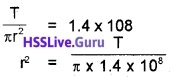r = 0.005m
∴ diameter = 0.01m.

Question 4.
The property of material bodies to regain its original size on the remaoval of deforming force is called elasticity.

1. What are the values of
• Young’s modulus for a perfectly rigid body
• Rigidity modulus of a liquid
2. Why do we prefer steel to copper in the manufacture of spring?
3. How much should the pressure on a littre of water be changed to compress it by 0.10%?
(B = 2.2 × 109N/m2)

1. The values:

• infinity
• Zero

2. Young’s modulus of steel is more than that of copper. Hence steel is more elastic than copper.

3.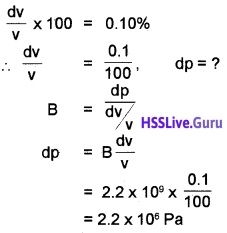### Plus One Physics Mechanical Properties of Solids NCERT Questions and Answers

Question 1.
A steel wire of length 4.7m and cross sectional area 3 × 10-5m2 stretches by the same amount as a copper wire of length 3.5m and cross sectional area of 4 × 10-5m2 under a given load. What is the ratio of the Young’s modulus of steel to that of copper?
For steel
l1 = 4.7m, a1 = 3 × 10-5m2
If F newton is the stretching force and ∆l metre the extension in each case, then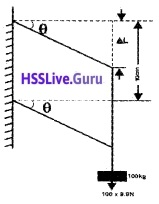For copper
l2 = 3.5m, a2 = 4 × 10-5m2Dividing (1) by (2), we getQuestion 2.
Following figure shows the strain-stress curve for a given material. What are

1. Young’s modulus and
2. approximate yield strength for this material?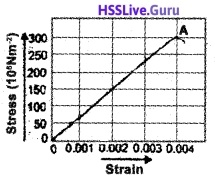1. It is clear from the graph that for a stress of 150 × 106Nm-2, the strain is 0.002
∴ Young’s modulus of the material= 7.5 × 1010Nm-2.

2. It is clear from the graph that A corresponds to the yield point. So, the approximate yield strength of them material is 300 × 106Nm-2 or 3 × 108Nm-2.

Question 3.
The edge of an aluminium cube is 10cm long. One face of the cube is firmly fixed to a vertical wal. A mass of 100kg is then attached to the opposite face of the cube. The shear modulus of aluminium is 25GPa. What is the vertical deflection of this face?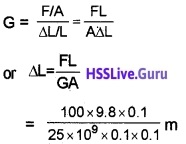3.92 × 10-7m
≃ 4 × 10-7m.

Question 4.
A steel cable with a radius 1.5cm supports a chair lift. If the maximum stress is not to exceed 108Nm-2, what is the maximum load the cable can support?
Maximum load = Maximum Stress × Cross sectional area
= 108Nm-2 × $$\frac{22}{7}$$ × (1.5 × 10-2)-2N
= 7.07 × 104N.

Question 5.
Compute the fractional change in volume of a glass slab when subjected to a hydraulic pressure of 10atm.